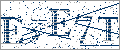>>

 Science >> Physics >> Phy-General >> Properties
Login or Register   Maxwell Boltzmann Distribution law
 New User RegisterLogin Member: Email: Password:
 Site SearchAdvance Search

Other animationsPotential and kinetic energyAnimation product List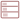Row format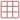Grid formatList formatOverview(For age - group : Above 16 )

Mawell Boltzmann's distribution law and graph is completely explained through animation. This 50-minute physics educational animation explains in detail the concept of molecular speed and energy distribution and is meant for high school and college physics classes.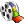Product - Animation
 Size/Time Subscribe Preview / Trailer Size (KB) 416 US\$    1.50 Rs. 108.00  Check price in your currency Subscription Days = 30 Watch a preview (opens in separate window) Time (hr:min:sec) 0:50:0 Add To Cart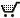Category : Physics Type : Animation Animation Type : Advanced Total animation length: 50 minutes Correlation between speed, KE, temperature Maxwell's formula Understanding distribution graph Case1 : Energy distribution and Temperature Interactive graph and molecular motion Daily life phenomenon - evaporation Daily life phenomenon - Chemical Reactions Case 2 : Energy distribution and molar mass Experimental verification of Maxwell's law Mathematical analysis of the experiment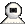Snapshots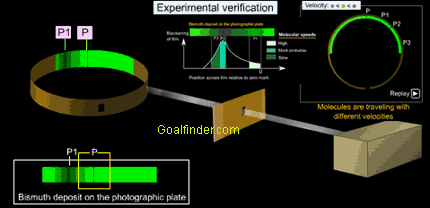Animation of experimental verification of Maxwell Boltzmann's distribution law using known concentrations of bismuth vapour and the velocities at different positions on the glass plate.Animated explanation of why do chemical reactions follow distribution law and how evaporation is affected by temperature and why it is a cooling process.Maxwell-Boltzmann distribution forms the basis of the kinetic theory of gases, and is useful for explaining molecular speed distribution, gas pressure, diffusion and evaporation of liquid.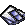Details of the animation/ movie /software
This science animation explains the following in detail:
1. Correlation between speed, KE, temperature : why does the speed of the molecules in a liquid or gas change continuously
2. Maxwell's formula
3. Understanding distribution graph : What does area under the curve , low tail, peak, most probable, average, rms speed, speed for lighter molecules
4. Case1 : Energy distribution and Temperature :To prove high percentage of molecules will have low energy at low temperatures.
5. Interactive graph and molecular motion : Through an interactive graph and increasing motion and collision of molecules understand the significance of graph
6. Daily life phenomenon - evaporation : High kinetic energy of the molecules is responsible for the phenomenon of evaporation
7. Daily life phenomenon - Chemical Reactions :
8. Case 2 : Energy distribution and molar mass : Speed of the molecules is inversely proportional to the molar mass of the molecules
9. Experimental verification of Maxwell's law : Experiment explains in details the rotating drum experiment and why spaced patterns of different densities are formed.
10. Mathematical analysis of the experiment
Others topics covered are :
• Why collisions cause a change in speed
• Conservation of KE
• Temperature is measure of average KE : importance of using statistical method for determining properties of large number of molecules.
• Why statistical methods are used for large number of molecules
• Why a bell shaped curve is produced?
• Maxwell Boltzmann graph
• most probable, average and rms speeds
• Escaping of hydrogen and helium, effusion & diffusion
• Understanding why particles position varies with speed?
• Explanatory Notes cover :
• Pre Maxwell's Theory
• How Maxwell developed the distribution law ?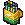Related Products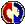Buyers Feedback:Q & A
 Q1: Why we use bismuth in above experiment? Surbhi 12/9/2015 Ans: Q2: fundamental mohd umair 8/1/2014 Ans: Q3: chemistry gas maxwel bolsman malsha thishakya 5/4/2009 Ans: We will appreciate if you as the question correctly Q4: what is the physical significance of maxwell-boltzman distribution/equation pushkar sasturkar 9/2/2009 Ans: Q5: what are the physical significance of three distributive laws? Sudipta Saha 7/1/2009 Ans: Q6: i want to understand the graph of molecular distribution Snehal Paladiya 5/12/2007 Ans: The graph shows the kinetic energy values and its popularity in molecules or simply how many particles in a system have a particular speed, for example the peak shows the most probable speed or the speed most likely to be possessed by any molecule in the system, however the distribution is not symmetrical about the peak. The speed or the Maxwell distribution depends on temperature and molar mass. The total curve represents speeds of all the molecules in the system.Give your comments